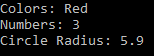1. Engineering
2. Computer Science
3. java programming introduction we are going to write a function...

# Question: java programming introduction we are going to write a function...

###### Question details

Java Programming

Introduction

We are going to write a function that utilizes generics

Function will look like:

public static <E extends Comparable<E>> E max (E [] list)

• This takes in a generic (E) that will extends the Comparable function (see last week's lecture)
• Remember these functions show how to compare two items
• Inside your code this allows access to the E.CompareTo()
• Return a generic (E)
• Function is named max
• Input is an array of generic type E

Objective:

• This function will find and return the maximum element in the array

Main Function

• Create an array of String, Integer and Double
• Strings String[] colors = {"Red","Green","Blue"};
• Integer[] numbers = {1, 2, 3};
• Double[] circleRadius = {3.0, 5.9, 2.9};
• Pass each to the max() function that you are writing and print the output:
• Example: System.out.println(max(colors));
• Will output “Red”
• Regardless of type, your function will find the maximum
• String, Integer and Double already extend Comparable

TIPS

• Review how to find the maximum of something in an array…
• Set maxElement to the first index
• Iterate in a for loop comparing the current element to maxElement
• If the current element of the array is greater than maxElement, update maxElement to that element
• Return maxElement
• How to do the comparison without knowing the type?
• You can call .CompareTo() which will return….
• Negative number if less than the parameter object
• Zero if equal to the parameter object
• Positive number if greater than the parameter object
• Hint: list[i].CompareTo(maxElement) > 0 will be a useful check inside your loop
• Copy the function exactly as presented
• public static <E extends Comparable<E>> E max (E [] list)
• You are not creating a class with generics
• Rather just a function that uses them
• Inside the function you can declare and make use of the E data type
• The E data type is a mystery to you
• But thanks to the .CompareTo() function you can figure out what the maximum element is in the array

Here is a sample output:###### Solution by an expert tutor